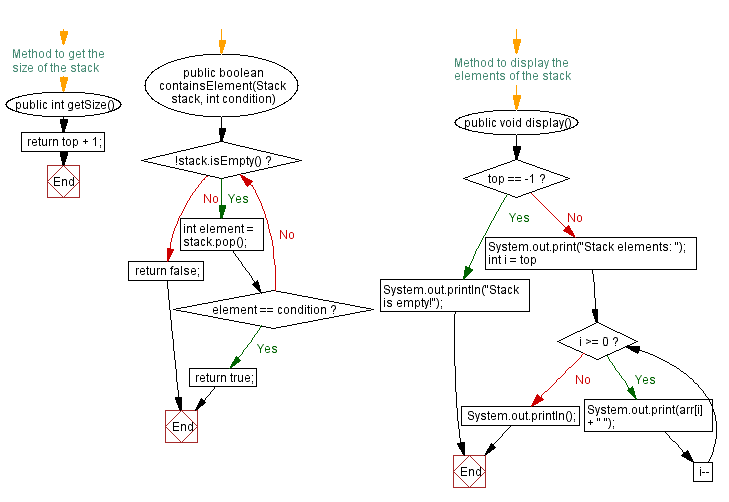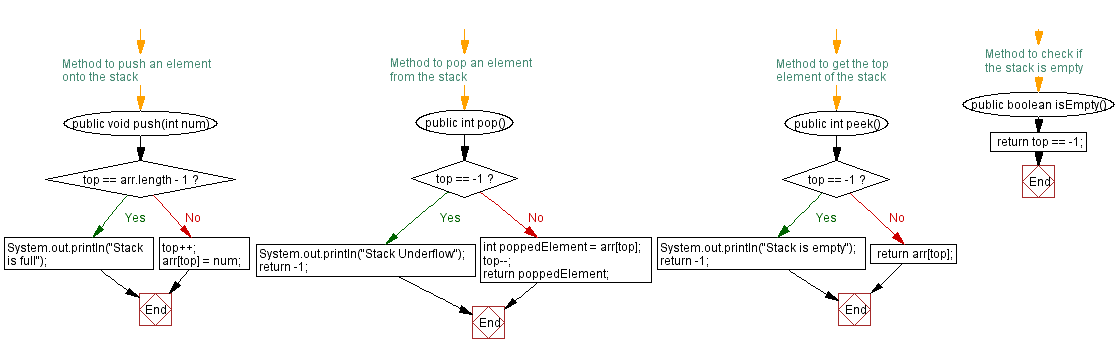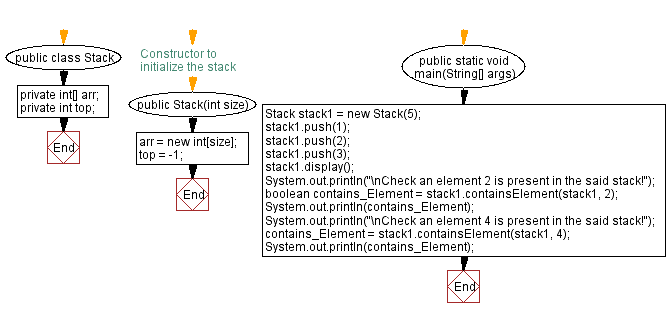# Java Exercises: Verify at least one element satisfy a condition

## Java Stack: Exercise-27 with Solution

Write a Java program that implements a stack and checks if at least one element of the stack satisfies a condition.

Sample Solution:

Java Code:

``````import java.util.*;
import java.util.function.Predicate;
public class Stack {
private int[] arr;
private int top;

// Constructor to initialize the stack
public Stack(int size) {
arr = new int[size];
top = -1;
}

// Method to push an element onto the stack
public void push(int num) {
if (top == arr.length - 1) {
System.out.println("Stack is full");
} else {
top++;
arr[top] = num;
}
}

// Method to pop an element from the stack
public int pop() {
if (top == -1) {
System.out.println("Stack Underflow");
return -1;
} else {
int poppedElement = arr[top];
top--;
return poppedElement;
}
}

// Method to get the top element of the stack
public int peek() {
if (top == -1) {
System.out.println("Stack is empty");
return -1;
} else {
return arr[top];
}
}

// Method to check if the stack is empty
public boolean isEmpty() {
}

// Method to get the size of the stack
public int getSize() {
}

public boolean containsElement(Stack stack, int condition) {
while (!stack.isEmpty()) {
int element = stack.pop();
if (element == condition) {
return true;
}
}
return false;
}

// Method to display the elements of the stack
public void display() {
if (top == -1) {
System.out.println("Stack is empty!");
} else {
System.out.print("Stack elements: ");
for (int i = top; i >= 0; i--) {
System.out.print(arr[i] + " ");
}
System.out.println();
}
}

public static void main(String[] args) {
Stack stack1 = new Stack(5);
stack1.push(1);
stack1.push(2);
stack1.push(3);
stack1.display();
System.out.println("\nCheck an element 2 is present in the said stack!");
boolean contains_Element = stack1.containsElement(stack1, 2);
System.out.println(contains_Element);
System.out.println("\nCheck an element 4 is present in the said stack!");
contains_Element = stack1.containsElement(stack1, 4);
System.out.println(contains_Element);
}
}
```
```

Sample Output:

```Stack elements: 3 2 1

Check an element 2 is present in the said stack!
true

Check an element 4 is present in the said stack!
false
```

Flowchart:Live Demo:

Java Code Editor:

Improve this sample solution and post your code through Disqus

Java Stack Previous: Verify all stack elements satisfy a condition.
Java Stack Exercises Next: Remove elements from a stack that do not meet a condition.

What is the difficulty level of this exercise?

Test your Programming skills with w3resource's quiz.

﻿